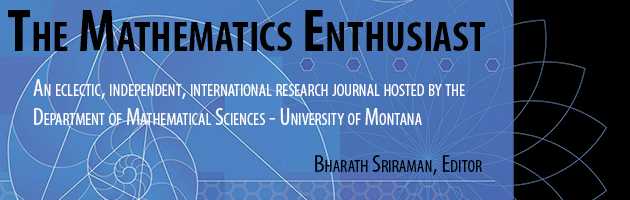•
•6

3

#### Abstract

Using 2x = 1 as an example, we discuss the cognitive load related to learning linear equation solving. In the framework of the Cognitive Load Theory we consider especially the intrinsic cognitive load needed in arithmetical, geometrical and real analytical approach to linear equation solving. This will be done e.g. from the point of view of the conceptual and procedural knowledge of mathematics and the APOS Theory. Basing on our observations, in the end of the paper we design a setting for teaching linear equation solving.

435

448

COinS